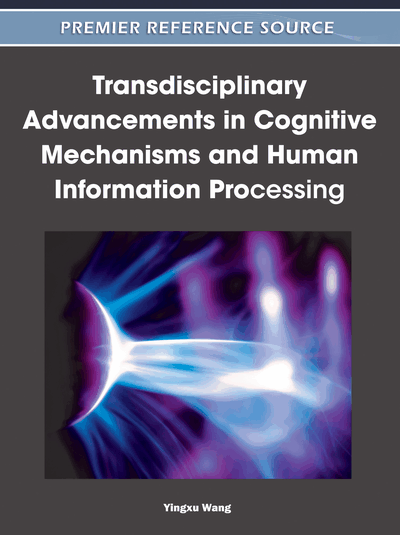# Advances in the Quotient Space Theory and its Applications

Liquan Zhao (Nanjing University of Finance and Economics and Anhui University, China) and Ling Zhang (Anhui University, China)
DOI: 10.4018/978-1-60960-553-7.ch015
Available
\$37.50
No Current Special Offers

## Abstract

Quotient space theory (QST), a new granule computing tool dealing with imprecise, incomplete and uncertain knowledge, uses a triplet, including the universe, its structure and attributes, to describe a problem space or simply a space. As one of important theories of granular computing (GrC), QST is very helpful to the study of cognitive informatics (CI). This article summarizes the quotient space’s model and its main principle. Then some basic operations on quotient space are introduced, and the significant properties of the fuzzy quotient space family are elaborated. Finally the main applications of quotient space theory are discussed.
Chapter Preview
Top

## The Model Of Quotient Space Theory And Its Main Features

The quotient space theory combines the different granularities with the concept of mathematical quotient set and represents a problem by a triplet (X, f, T). where X is the set of our discussing object, namely the universe; f(•) is the attribute function of universe X, and it may be multidimensional, f = (f1,...,fn), fi: X → Yi, which can be divided into two classes, the condition attribute and the decision attribute, denoted by the set C and D respectively, then f = C Υ D; T is the structure of universe X, namely the interrelations of elements.

## Complete Chapter List

Search this Book:
Reset